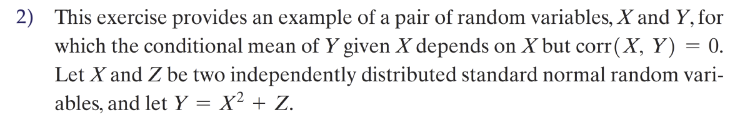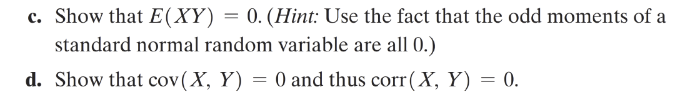Home / Expert Answers / Statistics and Probability / this-exercise-provides-an-example-of-a-pair-of-random-variables-x-and-y-for-which-the-conditional-pa413

# (Solved): This exercise provides an example of a pair of random variables, X and Y, for which the conditional ...This exercise provides an example of a pair of random variables, and , for which the conditional mean of given depends on but . Let and be two independently distributed standard normal random variables, and let . c. Show that . (Hint: Use the fact that the odd moments of a standard normal random variable are all 0 .) d. Show that and thus .

We have an Answer from Expert